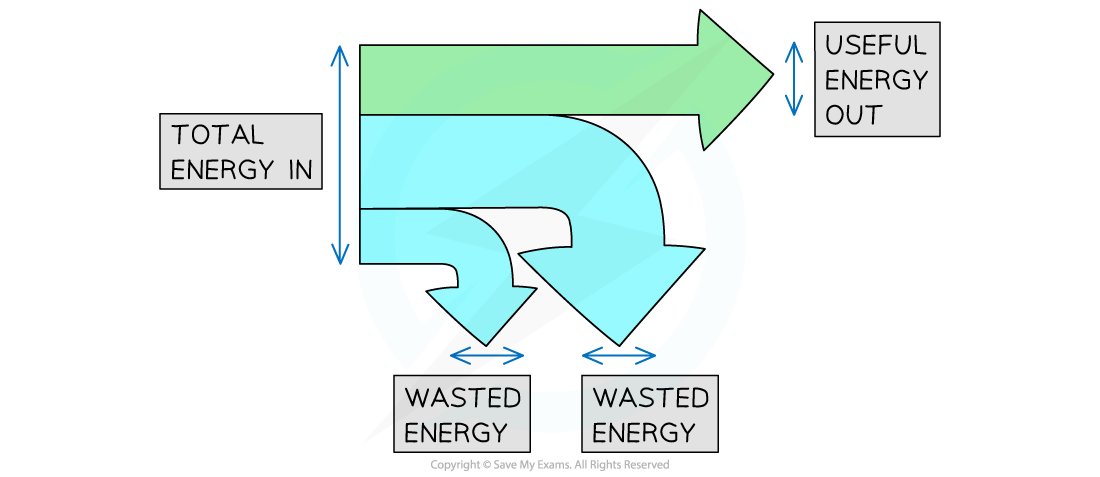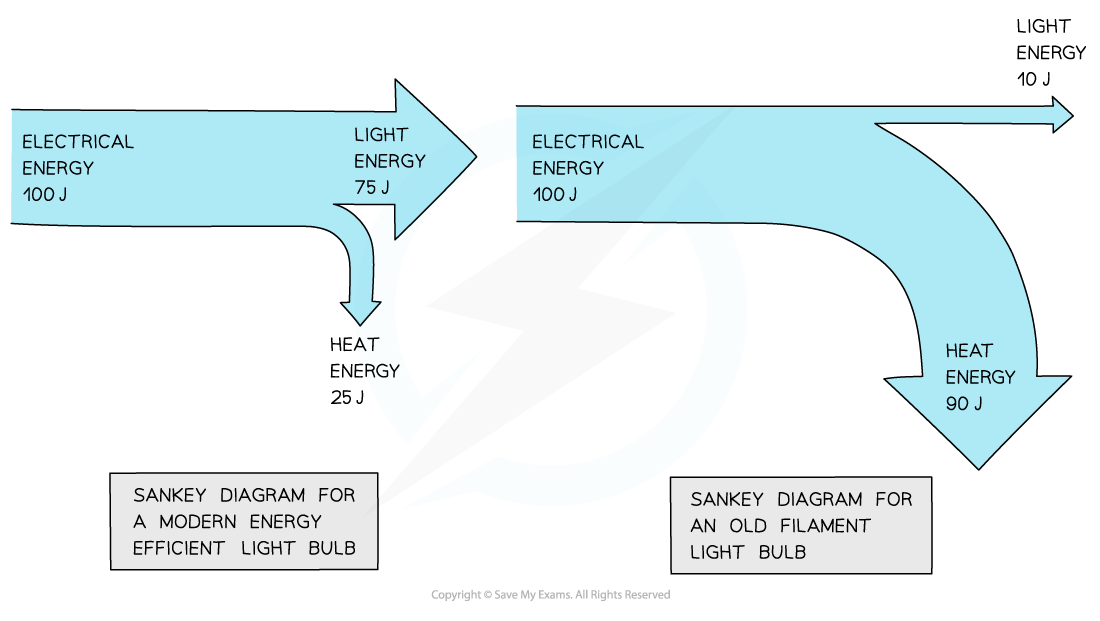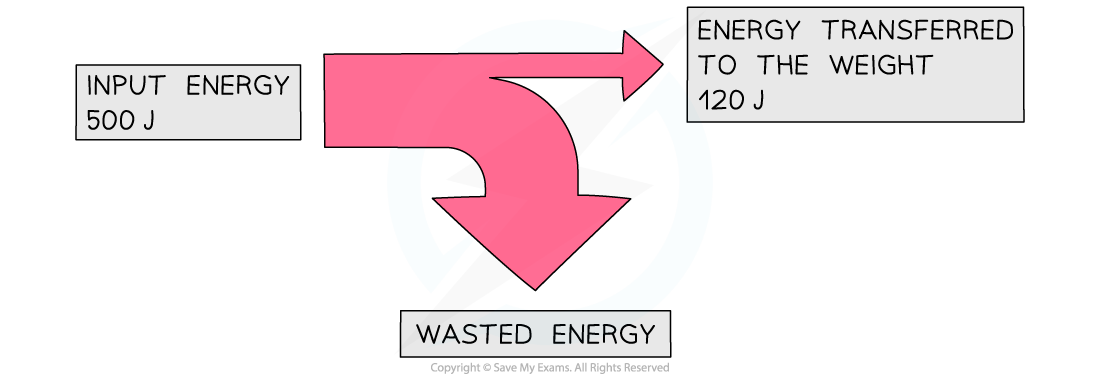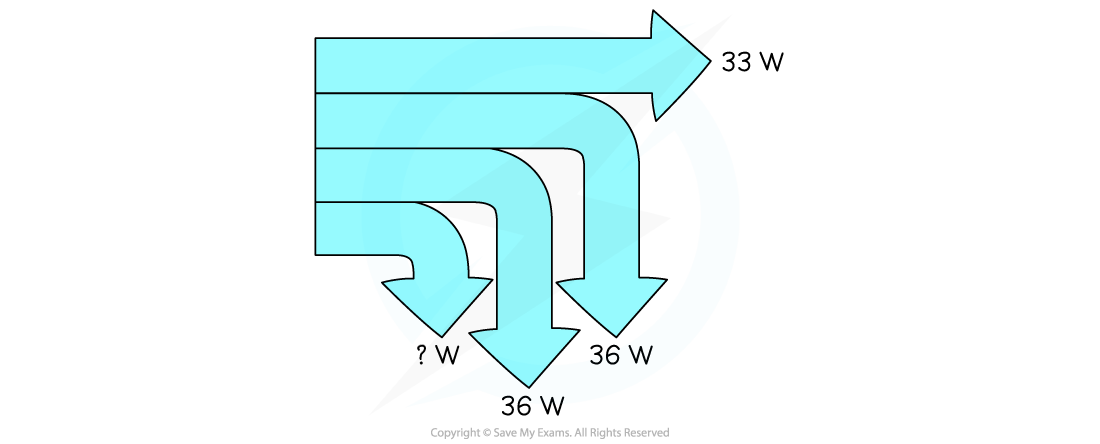# IB DP Physics: SL复习笔记8.1.2 Sankey Diagrams

### Sankey Diagrams

• Diagrams are used to represent energy transfers
• These are sometimes called Sankey diagrams
• The arrow in a Sankey diagram represents the transfer of energy:
• The end of the arrow pointing to the right represents the energy that ends up in the desired store (the useful energy output)
• The end(s) that point(s) down represents the wasted energyTotal energy in, wasted energy and useful energy out shown on a Sankey diagram

• The width of each arrow is proportional to the amount of energy going to each store
• As a result of the conversation of energy:

Total energy in = Useful energy out + Wasted energy

• A Sankey diagram for a modern efficient light bulb will look very different from that for an old filament light bulb
• A more efficient light bulb has less wasted energy
• This is shown by the smaller arrow downwards representing the heat energySankey diagram for modern vs. old filament light bulb

#### Worked Example

An electric motor is used to lift a weight. The diagram represents the energy transfers in the motor.How much energy is wasted?

Step 1: State the conservation of energy

• Energy cannot be created or destroyed, it can only be moved from one store to another
• This means that:

Total energy in = Useful energy out + Wasted energy

Step 2: Rearrange the equation for the wasted energy

Wasted energy = Total energy in – Useful energy out

Step 3: Substitute the values from the diagram

500 – 120 = 380 J

#### Worked Example

A small electric car is driven by a 120 Watt motor.

The useful power output of the motor is measured to be 33 W. While 36 W of power is wasted on friction losses and a further 36 W is wasted on traction losses.

Further power is lost by the electric car during operation. This situation is shown in the diagram below.Determine what is the remaining power loss for the electric car when operating?

Step 1: State the conservation of energy

• Energy cannot be created or destroyed, it can only be moved from one store to another
• This also applies to power
• This means that:

Total power in = Useful power out + Friction losses + Traction losses + Wasted power other losses

Step 2: Rearrange the equation for the wasted power

Wasted power other losses = Total power in – (Useful power out + Friction losses + Traction losses)

Step 3: Substitute the values from the diagram

120 – (33 + 36 + 36) = 15 W

#### Exam Tip

• Drawing good Sankey diagrams takes practice.
• Start by planning your diagram:
• How wide are you going to make the input arrow?
• How wide will the 'useful energy out' arrow need to be?
• How wide must the 'wasted energy' arrow be?
• Next, start drawing the diagram one step at a time:
• Draw the left hand side of the arrow, along with the line going across the top
• Next add the 'useful energy out' arrow, making sure it is the correct width
• Now carefully mark the start and end of the wasted arrow – make sure your marks are the correct distance apart!
• Finally join the markings together, finishing the 'wasted energy' arrow